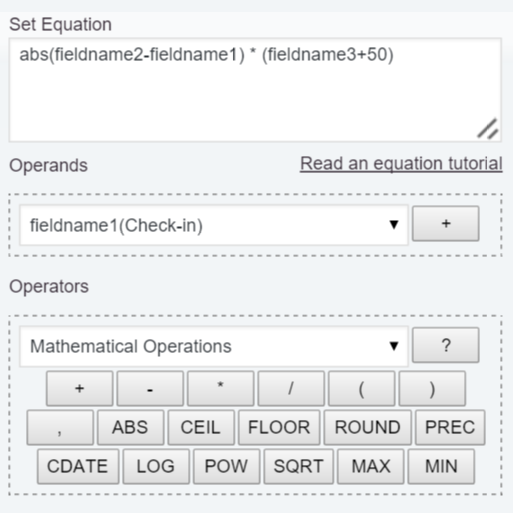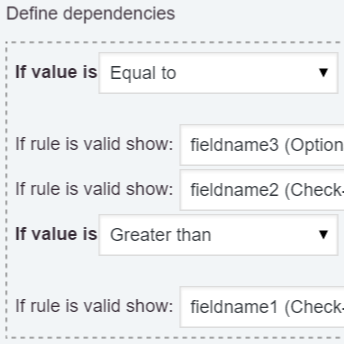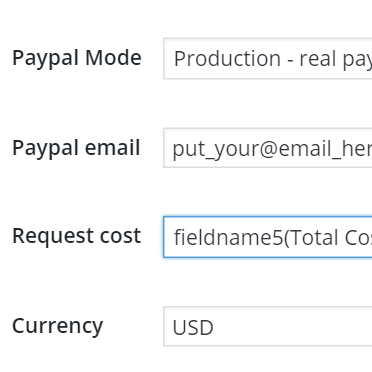# Features: Calculated Fields

The strongest and most versatile field of the plugin, essential for estimating product and service costs.

The plugin’s strength lies primarily in the Calculated Fields. A versatile field for calculating the price of products and services, the distance between addresses, areas, weight, time ranges, and more...

The calculated fields include multiple operation modules like: mathematical and logical operations, date-time operations, financial operations, and distance operations integrated with the Google Maps API.

Equation Editor### Equation Editor

The equation editor is an attribute of the calculated fields. The equation editor allows you to select the fields to be used in the equation (from a list of fields), and insert the operations that are available as buttons in the editor. The equation editor includes a list of operation modules (operation modules are limited only in the Developer version of the plugin).

Defining Dependencies### Defining Dependencies

The calculated fields allow dependencies to be defined according to their values. It is possible to display new fields if the equation result is less, equal, less than or equal to, bigger, or bigger than or equal to a number. The Calculated Fields can be used as auxiliary fields to create dependencies with common fields that do not support dependencies.

Request Cost### Calculated Field as PayPal Amount

If the calculated field is used to estimate the price of a product or service, it is possible to select the field to be submitted to PayPal and charge customers the estimated amount. Simply select the field from the list: "Request Cost" in the form settings. This attribute is also used by the WooCommerce add-on to estimate products prices.